﻿ Sample Applications - AustinMan Electromagnetic Voxels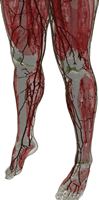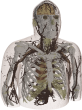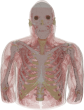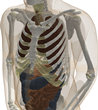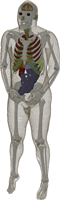# Sample Applications Using AustinMan and AustinWoman

## AustinMan Exposure to Plane Waves

F. Wei and A. E. Yılmaz, “A more scalable and efficient parallelization of the adaptive integral method—Part II: BIOEM application,” IEEE Trans. Antennas and Propag., vol. 62, no. 2, pp. 727-738, Feb. 2014.

• “The proposed method is used to compute the power absorbed by an anatomical human body model… The computations are performed for increasingly higher resolutions of the model and the power absorbed by the entire model and specific tissues in it are compared to safety standards.”
• “There is about 2.8-dB variation in the time-average power absorbed by the entire model as a function of the incident angle.”
• “The time-average power absorbed by the entire model is maximum when the wave is incident from the back.”
• “The results show that coarsest models can be used to estimate the total power absorbed by the AustinMan [v2.1] model within ±2% of the estimate from the finest model. The power absorbed in the localized tissues, however, show much more significant variations as the model is refined…”
• “For the skin, … the estimated power absorption increases significantly in the finer models … [the coarsest model has] about 20.4% of that in the highest fidelity model.”
• “The results show that highest-fidelity models should be used when estimating power absorbed by localized tissues.”

## Antennas Near Humans - Half-Wavelength Dipole

This section extends the results shown in the CS Bits & Bytes Newsletter.

### Problem

How much of the power radiated by a cell phone is absorbed in the human body? Where is this power absorbed?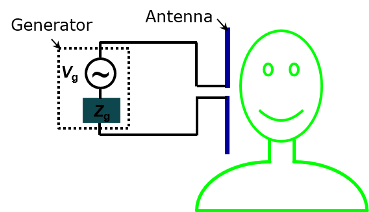### Established Health Effects of RF Heating

• “Studies on teratogenic effects of RF exposure… that cause heat stress in animal models have demonstrated that significant increases in the incidence of heat-induced abnormalities are seen at maternal temperature increases of approximately 2-2.5°C…”
• Teratogen - Any agent that can disturb the development of an embryo or fetus.
• Sterility can occur when mammalian testes, which are normally at a temperature of 33-35°C are heated… to temperatures approaching normal abdominal temperature (37-38°C)… Temporary sterility has been demonstrated in male rodents… lower sperm count and necrosis of testicular tissue was observed in testes heated to 39°C ”
• “… describe the effects on blood-brain-barrier (BBB) resulting from an exposure in combination with exposure to a virus or a drug… consistent with the weight of evidence demonstrating the BBB permeability is affected by RF exposures that cause a significant increase in brain temperature.”
• “… adverse effects of RF exposure of the eye, i.e. cataracts, are associated with significant temperature increases due to the absorption of RF energy.”

### Assumptions

• The generator (battery) voltage, impedance, and operating frequency are fixed.
• Generator impedance is conjugate matched to the input impedance of the antenna in free-space (far away from the human).

What we know:

• $V_\mathrm{g}$ (V): Peak generator voltage
• $Z_\mathrm{g}$ (Ω): Generator impedance
• $f$ (Hz): Operating frequency
• $P_\mathrm{s}=P_\mathrm{g}+P_\mathrm{abs}+P_\mathrm{rad}$

What we don't know:

• $P_\mathrm{s}$ (W): Total power supplied by the generator (time average)
• $P_\mathrm{g}$ (W): Power dissipated in the generator (time average)
• $P_\mathrm{abs}$ (W): Power absorbed by the body (time average)
• $P_\mathrm{rad}$ (W): Power radiated away (time average)
• $J$ (A/m): Surface current density on the antenna
• $Pd_\mathrm{abs}$ (W/m3): Power density in the body (time average)

What we actually modeled and solved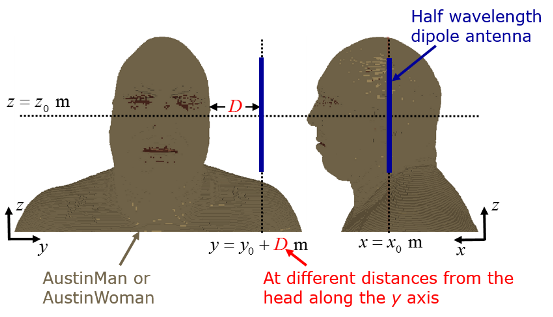### Methodology

#### Supercomputers

The list of top supercomputers in the world is available at top500.org.

These simulations were performed on Stampede at the Texas Advanced Computing Center which was ranked at #7 in the top500 in June 2014 and has >500,000 compute cores.

Typical simulation for this work (one antenna position): 1024 cores, 0.5 TB of memory, 2 hours wall-clock time (which would be 85 days on a single core).

#### Physics and Math

Maxwell's equations for electromagnetism:

$\nabla \times \overrightarrow{E}(\overrightarrow{r},t) = - \mu \frac{\partial\overrightarrow{H}(\overrightarrow{r},t)}{\partial t}$
$\nabla \times \overrightarrow{H}(\overrightarrow{r},t) = \frac{\partial\epsilon(\overrightarrow{r},t)*\overrightarrow{E}(\overrightarrow{r},t)}{\partial t} +\sigma(\overrightarrow{r},t)*\overrightarrow{E}(\overrightarrow{r},t)+\overrightarrow{J}(\overrightarrow{r},t)$
where $\overrightarrow{E}$ is the electric field strength, $\overrightarrow{H}$ is the magnetic field strength, $\epsilon$ is the electric permittivity, $\mu$ is the magnetic permeability, and $\sigma$ is the electric conductivity.

#### Scientific Software

FORTRAN 90 code used to perform the simulations (> 30,000 lines of code)

Scientific code libraries used: FFTW, LAPACK, and PETSc (each > 100,000 lines)

### Results

#### AustinMan and AustinWoman Simulations

The following parameters were used for the simulations for both models: $V_\mathrm{g}=1 \text{ V}$ and $f=900 \text{ MHz}$. The antenna's position was determined using $(x_0,y_0,z_0)=(0.18,0.425,1.74)\text{ m}$ for AustinMan and $(x_0,y_0,z_0)=(0.18,0.435,1.61)\text{ m}$ for AustinWoman.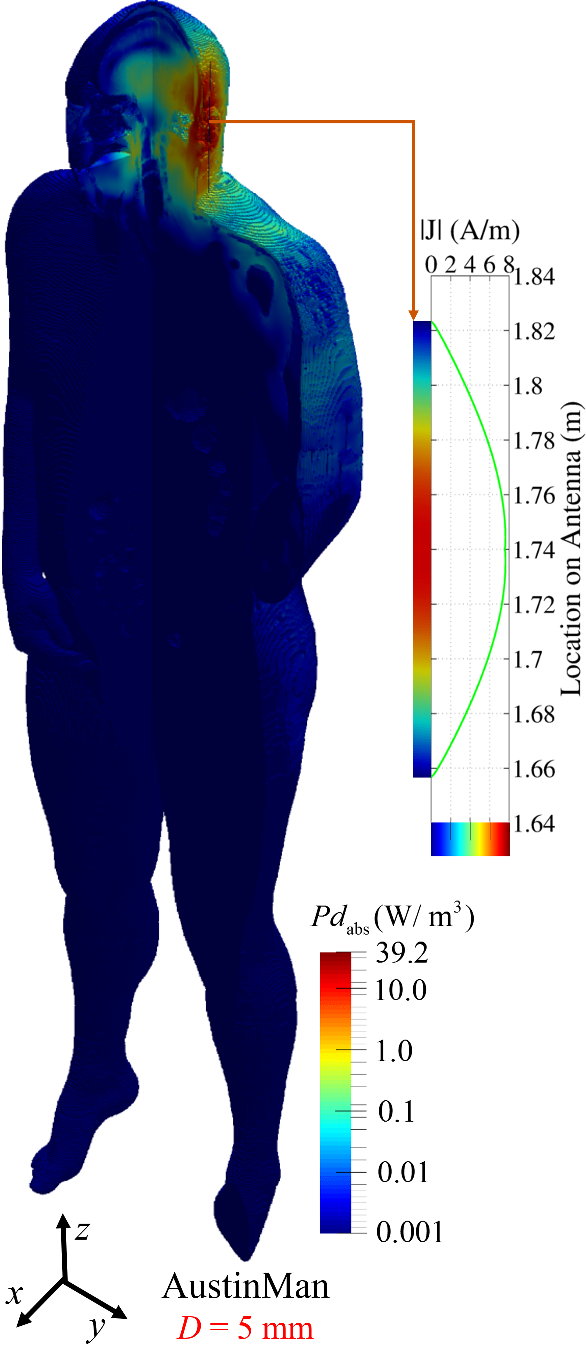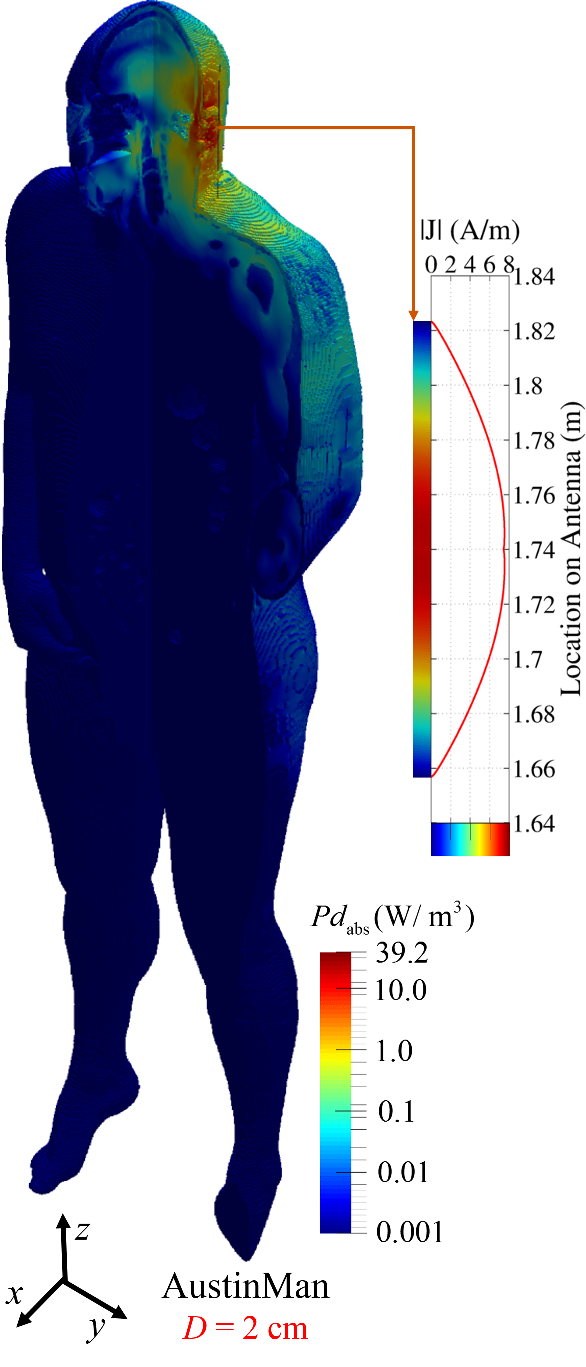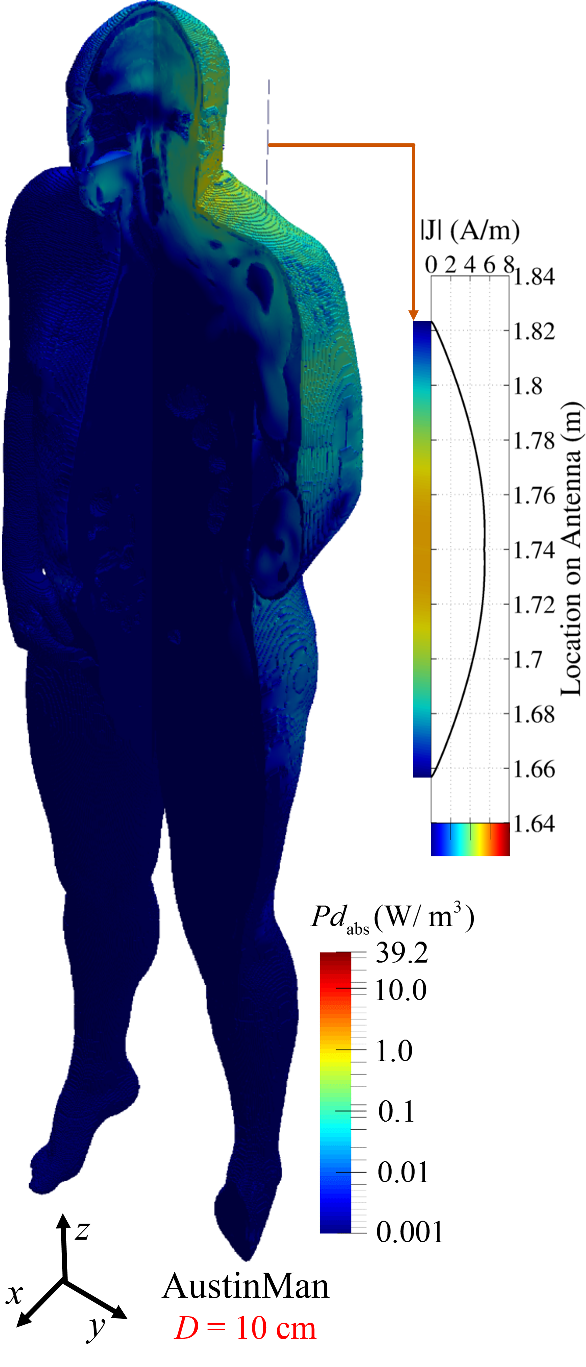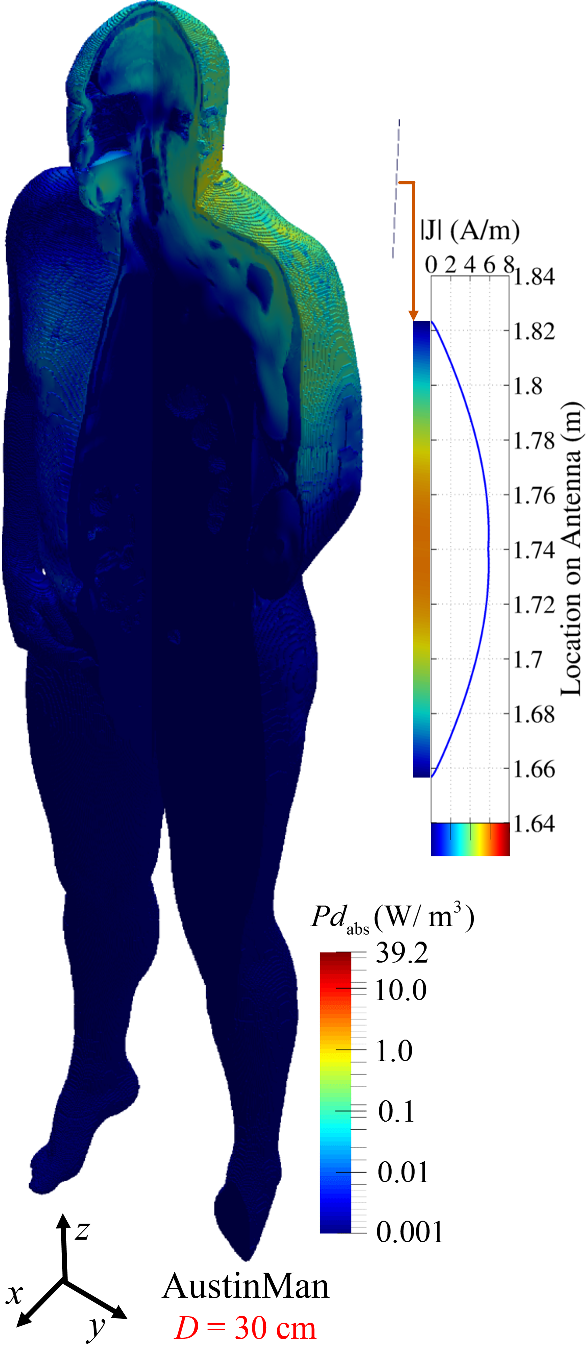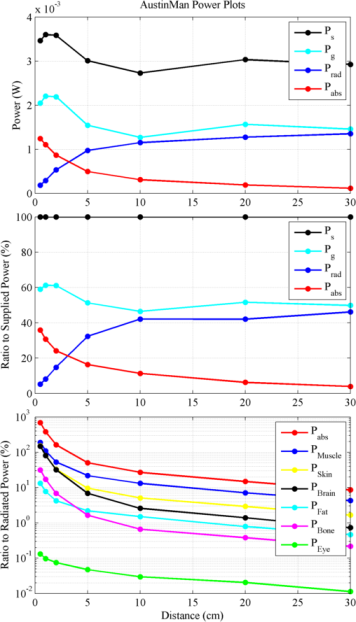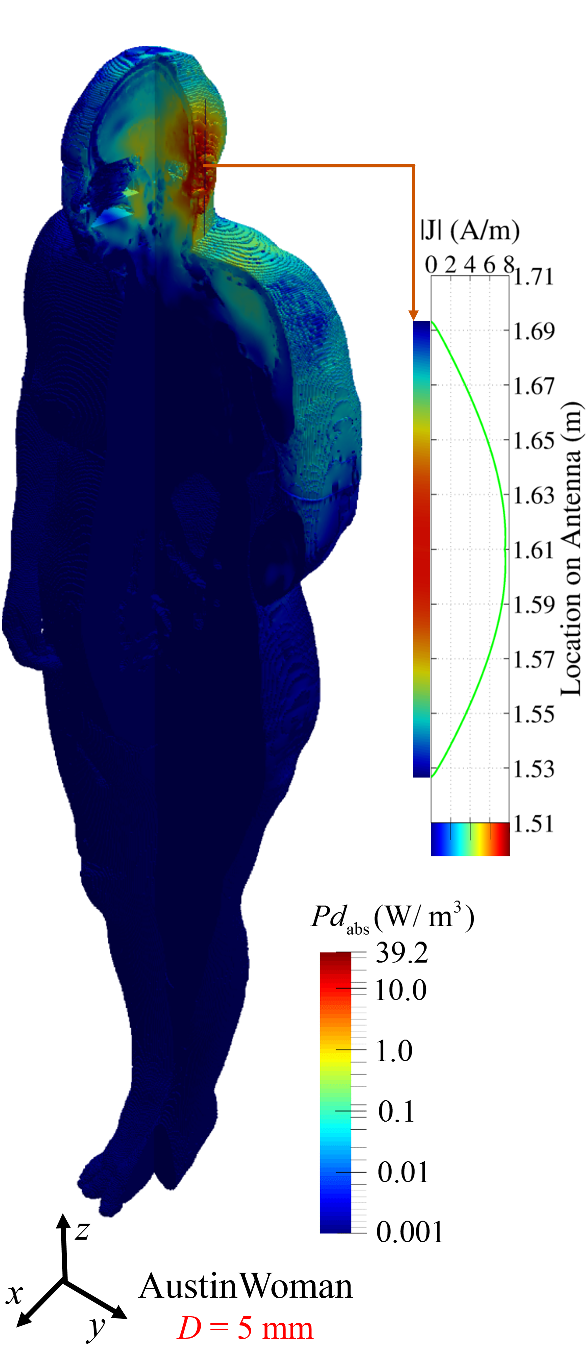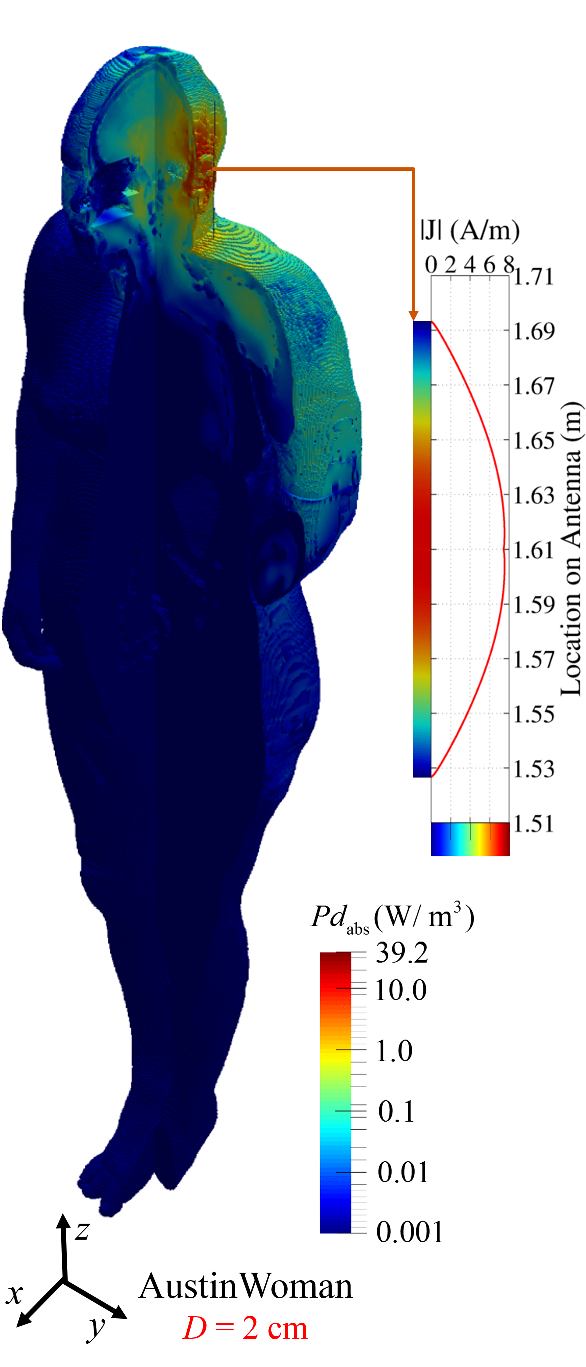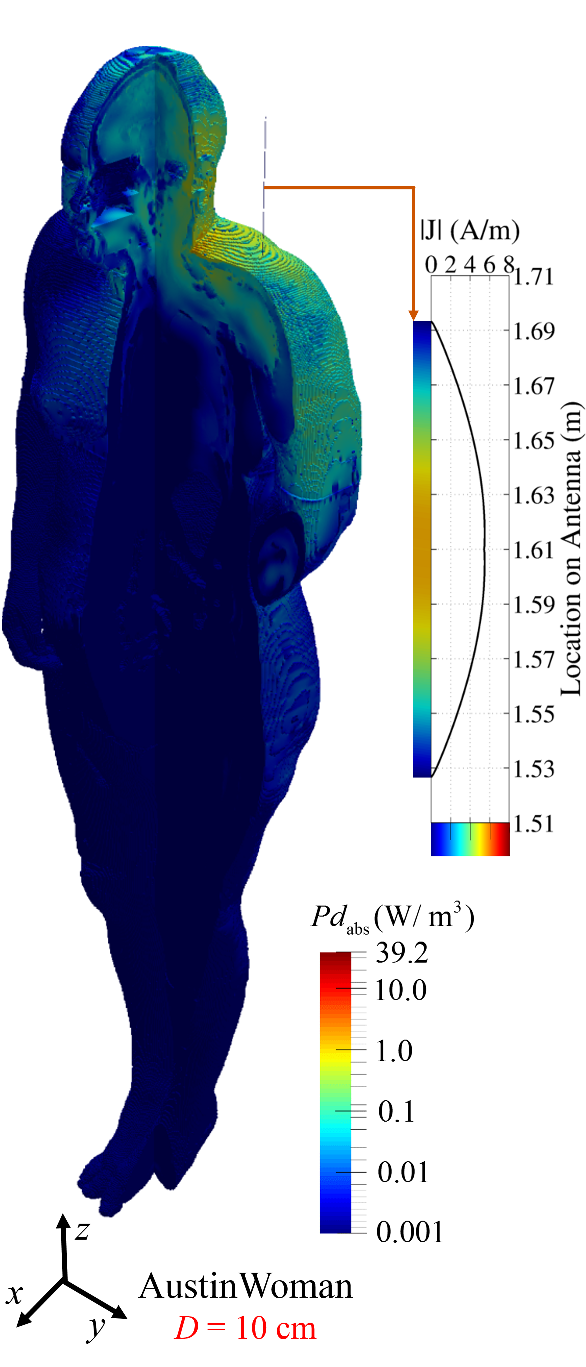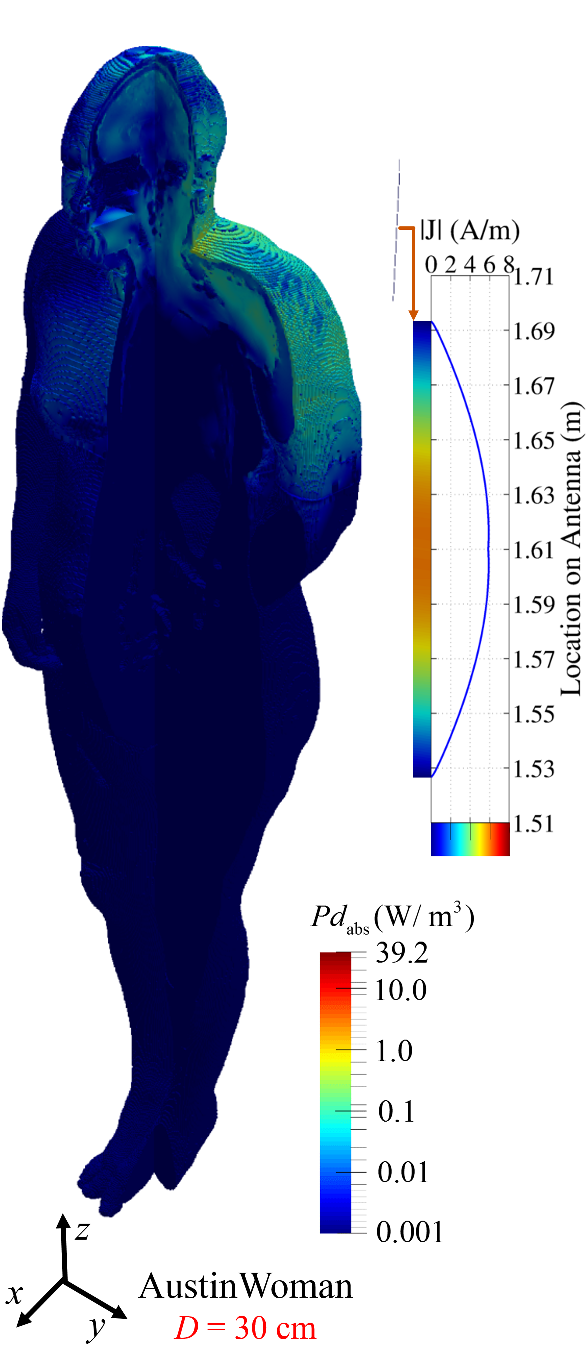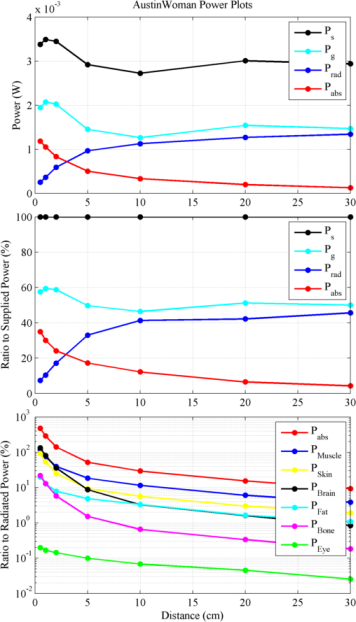AustinMan: AustinWoman: AustinMan: AustinWoman:

Observations:

• Total power supplied by the generator = Power dissipated in the generator (heats the device) + Power absorbed by the human body (heats the human) +  Power radiated away
• Total power supplied by the generator varies with phone location (human body loads and detunes the antenna).
• AustinMan: Minimum power is supplied when the antenna is 10 cm away from the head and ~32% more power is drawn from the generator when the antenna is 1 cm away from the head.
• AustinWoman: Minimum power is supplied when the antenna is 10 cm away from the head and ~28% more power is drawn from the generator when the antenna is 1 cm away from the head.
• The closer the device is to the head, the more power is absorbed by the human body and the less power is radiated away.
• AustinMan: At 5 cm, 16% of the supplied power is absorbed and 32% of it is radiated; at 5 mm, 36% of the supplied power is absorbed and 5% of it is radiated.
• AustinWoman: At 5 cm, 17% of the supplied power is absorbed and 33% of it is radiated; at 5 mm, 35% of the supplied power is absorbed and 7% of it is radiated.
• Muscle, skin, and brain tissues absorb most of the power.
• Fat absorbs relatively more power for AustinWoman when compared to AustinMan.
• When the antenna is 5 mm away from the head, more power is absorbed in the muscle, skin, and brain tissues than is radiated away (~1.5x-2x the radiated power is absorbed in each of these tissues).
• When the antenna is more than 20 cm away from the head, the total supplied, dissipated, absorbed, and radiated powers all vary slowly.